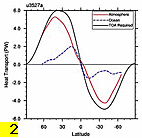f.e15.F2000.f09_f09.pda.79
and
f.e15.F2000.f09_f09.pia.79

DIAG Set 2 - Line plots of annual implied transports

The computation of the implied northward transports follows the conventions described
in the paper by Trenberth and Caron (2001). Their corrections applied to the southern
oceans poleward of 30S are not used in the calculations, and the NCEP derived values
plotted here are their unadjusted values. Webpage about the NCEP derived data.
A plot of the ocean basins used in the calculations.

Annual Implied Northward Transports
Ocean Heat plot
Atmospheric Heat plot
Ocean Freshwater plot
Surface Heat plot